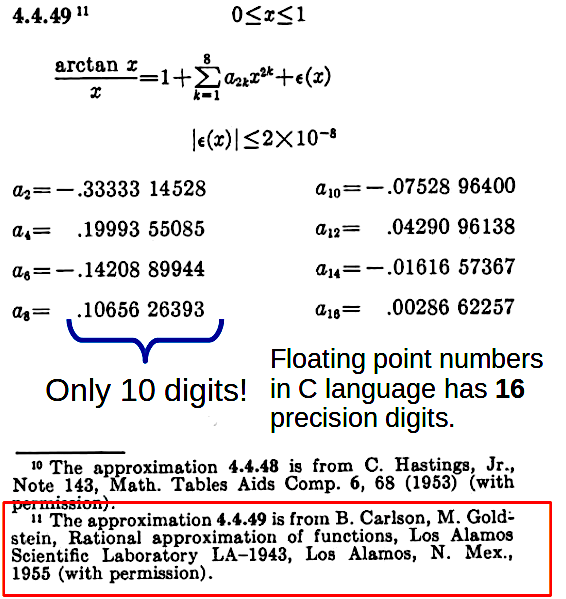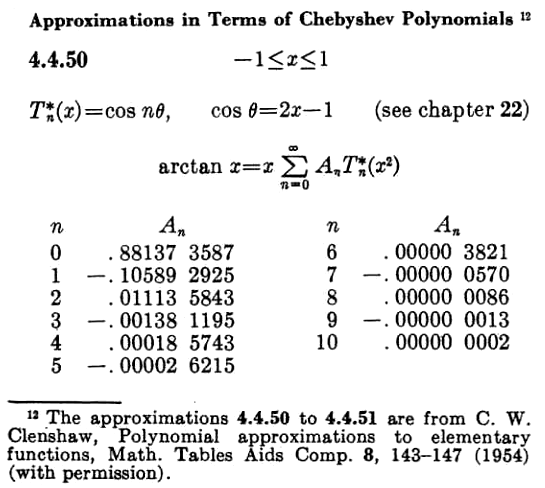# Introduction

The second programming language I ever learned was the C language. About the first half of the ’80s, I got The C Programming Language book . Well written, easy to understand, I Love it. At that time, a friend of mine in college gave me a copy of “Lattice C,” a C compiler, to run it in the DOS command line, of course. At that time internet didn’t exist; to browse some books, you had to go to a library. With the C compiler, I practiced the exercises in the book.

However, something was missing. The compiler came without a mathematical library to compute sine, cosine, exponential function, etc. Those are called elementary transcendental functions. I was studying Mechanical Engineering at that time, and those functions were vital to solving college problems. Those days I had a part-time job teaching the Basic language to the owners of the ZX81 (Times Sinclair) home computer. The folks that hired me for the classes gave me the ZX81 manual. I asked them if they had something about the computing of those transcendental functions. Fortunately, they have it. They lent me a book describing the ZX81 ROM where there was an explanation about the source of the implementation of their transcendental functions.

I found that those transcendental functions, calculated by the ZX81 ROM, were using formulas from the book “Handbook of Mathematical Functions by Abramowitz and Stegun” . Which eventually, I found it in the School of Mathematics Library at my college. Finally! I had the formulas referenced by the ROM book!Figure 0.1: All the approximations in the Handbook of Mathematical Functions has 10 or less digits of precision.

Unfortunately, those formulas in the book have just ten digits of precision, and in C language, I had double-precision arithmetic with 16 precisión digits. I had to find a way to compute the coefficients in those formulas, but this time with 16 precision digits. Nonetheless, that was not an easy task. The maths behind the coefficients’ computation were complicated and valuable enough that the handbook gives the authorship attribution to the Los Alamos Scientific Laboratory and has to ask permission to publish the Chebyshev approximation from Clenshaw . Another hint that they were not easy is that they had numerical form only, and they were from the 1950’.

Long story short, I had to evaluate growingly complicated integrals over the so-called Chebyshev Polynomials to get the coefficients. In the end, for some transcendental functions, I could find analytical solutions for these integrals and get the desired approximation.

The beauty of the analytical form is that you can have arbitrary precision. You are limited only by the accuracy of the arithmetic you use. In the code below, we shall test the $$atan$$ approximation using 30 precision digits.

For $$atan$$, the inverse of the tangent function, I found the following approximation:

\begin{align} atan(x) &= \sum_{k=1}^\infty b_k·P_k(x) ~, ~~~\text{for}~ \lvert x \rvert \leq 1 \\ b_k &= (-1)^{(k-1)}\, (\frac{2}{2\,k-1}) \, (\sqrt{2}-1)^{(2k-1)} \\[5pt] P_{0}(x) &= x \\ P_{1}(x) &= x \\ P_{k}(x) &= (4\,x^2-2)·P_{k-1} - P_{k-2} \end{align}

We only need the squared root of $$2$$ and arithmetic to compute the $$atan$$ function with these formulas. The Handbook has the values of some irrational numbers with a lot of digits of precision, including $$\sqrt{2}$$.

from mpmath import mp, mpf
mp.dps = 30 # Number of precision digits

def atan_poly_cheb(x):
atan_poly_cheb.p2 = 4*x*x-2
atan_poly_cheb.p_nm1 = x
atan_poly_cheb.p_nm2 = x
yield x
while True:
p_np1 = atan_poly_cheb.p2*atan_poly_cheb.p_nm1 \
- atan_poly_cheb.p_nm2
atan_poly_cheb.p_nm2 = atan_poly_cheb.p_nm1
atan_poly_cheb.p_nm1 = p_np1
yield p_np1

We will use Python code to test this approximation works as intended. The polynomials $$P_k(x)$$ are computed with the atan_poly_cheb(x) function, and the $$b_k$$ coefficients with atan_coef(). Both are Python generators, so you can call them as many times as you want and get as many values you need. We use the mpmath module to compute $$atan$$ with 30 precision digits.

def atan_coef():
SQRT2_M1 = mp.sqrt(2) - mpf(1)
SQRT2_M1_2 = SQRT2_M1*SQRT2_M1
atan_coef.k = mpf(0)
atan_coef.rho_factor = mpf(-2)/SQRT2_M1

while True:
atan_coef.k += mpf(1)
atan_coef.rho_factor *= - SQRT2_M1_2
coef = atan_coef.rho_factor/(2*atan_coef.k-1)
yield coef

The function mpf(x), in the mpmath module, creates a floating point number with multi-precision, 30 digits in our case. Then the arithmetic with these numbers maintains that precision. The computation of $$b_k$$ is made in a iterative way:

\begin{align} rho\_factor_0 &= -2/(\sqrt{2}-1) \\ rho\_factor_k &= - rho\_factor_{k-1}\times(\sqrt{2}-1)^2 \\[3pt] b_k &= \frac{rho\_factor_k}{2\,k-1} \end{align} The atan_approx(x) function call the coefficient and Chebyshev polynomial generators, and with 37 of them compute the $$atan$$ function with 30 digits of precision. This is about 1.2 terms per precision digit. For 16 digits, double-float precision, you only need 19 of them.

def atan_approx(x):
result = 0
cheby = atan_poly_cheb(x)
coef = atan_coef()

for i in range(37):
result += next(coef) * next(cheby)

return result

We evaluate the approximation for $$x=1$$ where the $$atan(1)$$ is $$\pi/4$$. We multiply the result by $$4$$ and compare it with the ground truth value of $$\pi$$ provided by the mpmath module. Also we evaluate $$atan$$ for a random number in $$[-1,1]$$ and compare the result, again with the value according to mpmath.

def main():
pi_approx = atan_approx(1)*4
print("*** Approximation to pi ****")
print("Pi Approx: ", pi_approx)
print("Pi Exact : ",mp.pi)
print("Pi Approx Error: ", pi_approx-mp.pi)

from random import random
x = mpf(random()*2 - 1)
approx = atan_approx(x)
exact = mp.atan(x)

print("\n*** atan of a random number ****")
print("x: ", x)
print("Approx atan: ", approx)
print("Exact atan : ", mp.atan(x))
print("Atan Approx Error: ", approx-exact)
*** Approximation to pi ****
Pi Approx:  3.14159265358979323846264338328
Pi Exact :  3.14159265358979323846264338328
Pi Approx Error:  3.54987407349455312435277854377e-30

*** atan of a random number ****
x:  -0.817895132505307209669354051584
Approx atan:  -0.685557759217410550765244311608
Exact atan :  -0.685557759217410550765244311608
Atan Approx Error:  0.0

At the end we achieve the 30 digits precision, showing the approximation works.

In this post we will introduce the Chebyshev Approximation, and show you how did I found the one for the $$atan$$ function. I hope you like it.

# 1 The Chebyshev Approximation

You can approximate a function with the following formulas:

\begin{align} f(x) &\approx \frac{a_0}{2} + a_1·T_1(x)+a_2·T_2(x)+ \dots a_p·T_p(x) \tag{1.1} \\[3pt] a_k(f) &= \frac{2}{\pi} \int_{-1}^1 \frac {f(x) · T_k(x) }{\sqrt{1-x^2}} dx \tag{1.2} \end{align}

Where $$T_k(x)$$ are the Chebyshev polynomials of the first kind:

\begin{align} T_0(x) &= 1 \\ T_1(x) &= x \\ T_2(x) &= 2x^2-1 \\ T_{n+1}(x) &= 2x·T_{n}(x)-T_{n-1}(x) \\ T_{n+2}(x) &= (4x^2-2)·T_{n}(x)-T_{n-2}(x) \end{align}

The trick with these polynomials is that if $$x=cos(\theta)$$ then $$T_k(x)=cos(k\, \theta)$$. In other words, you can express $$cos(k\,\theta)$$ as a polynomial of $$cos(\theta)$$. For example, the trignometric identity $$cos(2\,\theta)=2·cos^2(x)-1$$ means that if we do the change of variable $$x=cos(\theta)$$, then $$T_2(x)=cos(2\,\theta)=2x^2-1$$.

If in (1.2) we make the change of variable $$x=cos(\theta)$$, and substitute $$T_k(x)=cos(k\, \theta)$$, we get:

\begin{align} a_k(f) &= \frac{2}{\pi} \int_0^\pi {f(cos(\theta))·cos(k~\theta) } \,d\theta \tag{1.3} \end{align}

### An Odd function

In math when $$f(-x)=f(x)$$ you say that $$f$$ is even, and if $$f(-x)=-f(x)$$ then $$f$$ is odd. The $$T_k$$ polynomials for k even, contain only even powers of $$x$$, and for $$k$$ odd only odd powers of $$x$$; which causes $$T_k(x)$$ is an even function when $$k$$ is even, and odd when $$k$$ is odd.

If we split the integration interval in (1.2), and make a change of variable to get both integrals in $$[0,1]$$:

\begin{aligned} a_k(f) &= \frac{2}{\pi} \int_{-1}^1 \frac {f(x) · T_k(x) }{\sqrt{1-x^2}} dx \\ &= \frac{2}{\pi} \int_{-1}^0 \frac {f(x) · T_k(x) }{\sqrt{1-x^2}} dx + \frac{2}{\pi} \int_0^1 \frac {f(x) · T_k(x) }{\sqrt{1-x^2}} dx \\ &= \frac{2}{\pi} \int_0^1 \frac {f(x) · T_k(x) + f(-x) · T_k(-x) }{\sqrt{1-x^2}} dx \end{aligned}

Now we can see for $$f$$ even and $$k$$ odd $$f(-x)·T_k(-x) = -f(x)·T_k(x)$$ and $$a_k(f)$$ vanishes; the same happens when $$f$$ is odd and $$k$$ is even. In summary, we can say:

\begin{aligned} \text{When } f ~\text{is even:} & \\ a_k(f) &= \begin{cases} a_k(f)\neq 0 & \text{if } k ~\text{is even,} \\ 0 & \text{if } k ~\text{is odd} \end{cases} \\ \text{When } f ~\text{is odd:} & \\ a_k(f) &= \begin{cases} 0 & \text{if } k ~\text{is even,} \\ a_k(f)\neq 0 & \text{if } k ~\text{is odd} \end{cases} \end{aligned}

## 1.1 Approximation using the Derivative of the Function

Sometimes it is easier to find the approximation using the function first derivative instead of the function itself. To find the required formula, we will use the Integration by Parts rule, which you can remember from its wikipedia article.

\begin{aligned} cos(k\,\theta) &= \frac{d(sin(k\,\theta)/k)}{d\theta}, \\ a_k(f) &= \frac{2}{\pi} \int_0^\pi {f(cos(\theta))·cos(k\,\theta) } \,d\theta \\ \implies \\ &= \frac{2}{\pi} f(cos(\theta))·\frac{sin(k\,\theta)}{k} \Big|_0^\pi + \frac{2}{k\,\pi} \int_{0}^\pi f'(cos(\theta))·sin(\theta)·sin(k\,\theta) \,d\theta \\ a_k(f) &= \frac{2}{k\,\pi} \int_0^\pi f'(cos(\theta))·sin(\theta)·sin(k\,\theta) \,d\theta \end{aligned}

Now we will go to the polynomial form by changing $$x=cos(\theta)$$:

\begin{align} a_k(f) &= \frac{2}{k\,\pi} \int_{-1}^1 \frac{f'(x)·sin(k\,\theta)·sin(\theta)}{\sqrt{1-x^2}}\,dx \\ &= \frac{2}{k\,\pi} \int_{-1}^1 \frac{sin(k\,\theta)}{sin(\theta)} · f'(x)·\sqrt{1-x^2} \,dx \tag{1.4} \end{align}

Where the quotient $$\frac{sin(k\,\theta)}{sin(\theta)}$$ in (1.4) are the Chebyshev polynomials of the second kind $$U_k$$.

\begin{align} U_0(x) &= 1 \\ U_1(x) &= 2x \\ U_2(x) &= 4x^2 - 1 \\ U_{n+1}(x) &= 2x·U_n(x)-U_{n-1}(x) \\ U_{n+2}(x) &= (4x^2-2)·U_n(x)-U_{n-2}(x) \end{align}

The quotient $$\frac{sin(k\,\theta)}{sin(\theta)}$$ in (1.4), for $$k=2$$ is $$2·cos(\theta)=2\,x$$, which es equal to $$U_1(x)$$; that means we should replace that quotient with $$U_{k-1}$$.

$a_k(f) = \frac{2}{k\,\pi} \int_{-1}^1 U_{k-1}(x) · f'(x)·\sqrt{1-x^2} \,dx \tag{1.5}$

# 2 Approximation to atan

For the inverse of the tangent function $$atan$$, we can get its series expansion by using sageMath online:

var('x')
f = atan(x)
f.taylor(x, 0, 7)

$atan(x) = x - \frac{x^3}{3} + \frac{x^5}{5} - \frac{x^7}{7} \tag{2.1}$

In the graph “atan function plot” 2.1, we can see $$atan(x)$$ is an odd function. Which is also something we can notice from its Taylor series. Therefore, the $$atan$$ approximation will only have odd $$a_k$$ terms, e.g. $$a_1, a_3, ~\dots$$. Which, by the way, correspond to even polynomials $$U_0, U_2, \dots$$. Another useful feature from the Taylor series is the alternating sign of the terms.